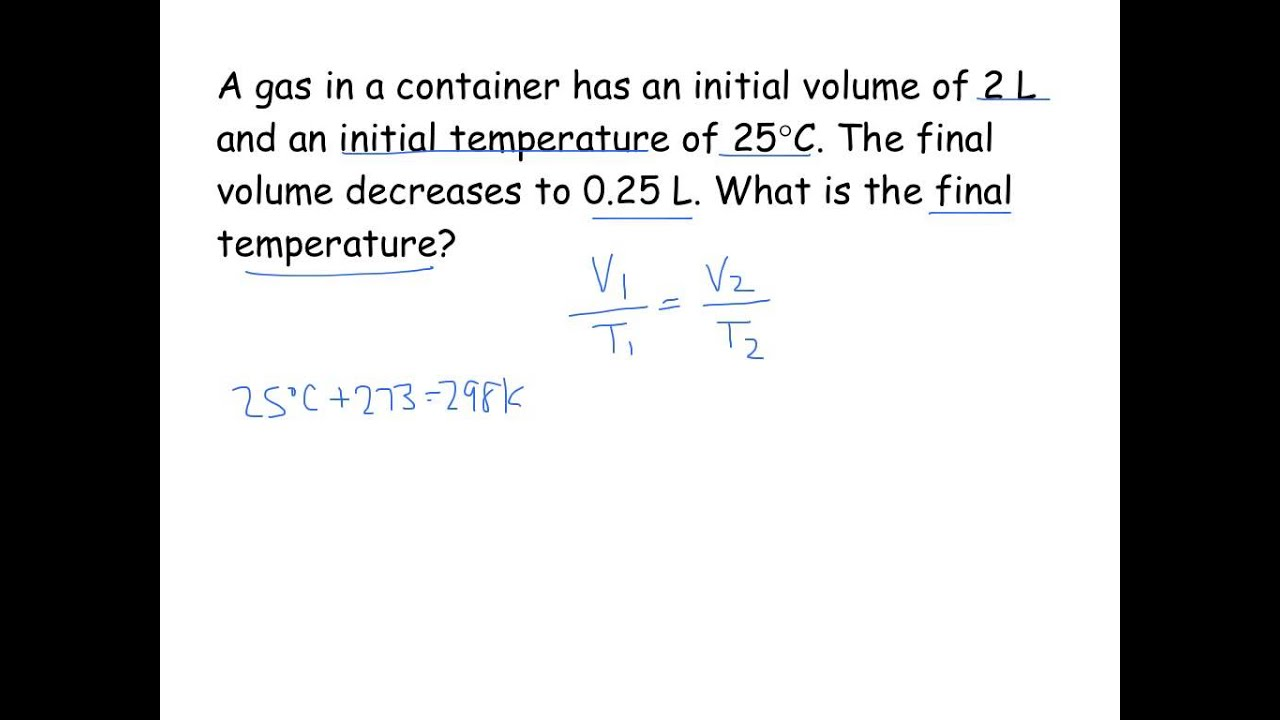Charles Law vs Boyle law

Charles’ law and Boyle’s law are two very important laws concerned with gases. These two laws can describe many properties of the ideal gases. These laws are widely used in fields such as chemistry, thermodynamics, aviation and even military applications. It is vital to have a solid understanding in these two laws in order to excel in such fields. In this article, we are going to discuss what Charles’ law and Boyle’s law are, their definitions, applications of Charles’ law and Boyle’s law, their similarities, and finally the differences between Charles’ law and Boyle’s law.

Boyle’s Law

Boyle’s law: The pressure exerted by a gas results from the impact of its molecules on the walls of the container. The collision rate, or the number of molecular collisions with the walls per second, is proportional to the number density of the gas.

Boyle’s law is a gas law. It is defined for an ideal gas. A proper understanding about ideal gas is necessary, to understand these ideal gas laws. Ideal gas is a gas for which the volume occupied by each molecule is zero; also the intermolecular attractions between the molecules are zero. Such ideal gases do not exist in real life conditions. The gases, which exist in real life, are known as real gasses. Real gases have molecular volumes and intermolecular forces. If the combined volume of all molecules of a real gas is negligible compared to the volume of the container, and the intermolecular forces are negligible compared to the velocities of the molecules, then the gas can be considered an ideal gas in that system. The Boyle’s law, which was proposed in 1662 by the chemist and physicist Robert Boyle, can be stated as follows. For a fixed amount of an ideal gas, kept at a fixed temperature, pressure and volume are inversely proportional.

A closed system is a system where no mass interchange between the surrounding and the system is possible, but energy exchange is possible. The Boyle’s law suggests that the product of the pressure and the volume of an ideal gas, in a constant temperature, to be constant. In other words, P V = K, where p is the pressure, V is the volume, and K is the constant. This means, if the pressure of such a system is doubled, the volume of that system becomes half of its original value.Charles’ Law

Charles law is also a gas law, which is defined to an ideal gas in a closed system. This states that for a closed ideal gas system under constant pressure, the volume of the system is directly proportional to the temperature of the system. This law was first published by the French philosopher Joseph Louis Gay-Lussac, but he credited the discovery to Jacques Charles. This law suggests that for such a system, the ratio between the temperature and the volume must be a constant. In other words, V/T = K, where V is the volume of the gas and T is the temperature of the gas. It must be noted that mathematically, this proportionality will only work for Kelvin scale, which is an absolute temperature scale.

 What is the difference between Charles’ law and Boyle’s law?• Charles’ law is defined for a system with a constant pressure while Boyle’s law is defined for a system with constant temperature. • The two terms involved in Charles’ law are directly proportional to each other while the terms involved in Boyle’s law are inversely proportional.

### Related posts:• Slide 1